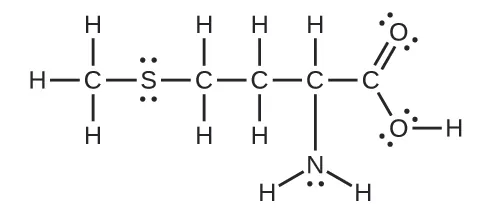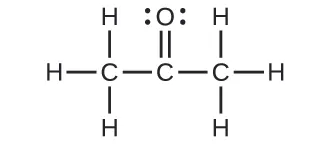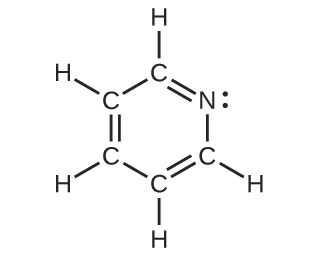Chemistry: Atoms First

# Exercises

Chemistry: Atoms FirstExercises

### 5.1Valence Bond Theory

1.

Explain how σ and π bonds are similar and how they are different.

2.

Use valence bond theory to explain the bonding in F2, HF, and ClBr. Sketch the overlap of the atomic orbitals involved in the bonds.

3.

Use valence bond theory to explain the bonding in O2. Sketch the overlap of the atomic orbitals involved in the bonds in O2.

4.

How many σ and π bonds are present in the molecule HCN?

5.

A friend tells you N2 has three π bonds due to overlap of the three p-orbitals on each N atom. Do you agree?

6.

Draw the Lewis structures for CO2 and CO, and predict the number of σ and π bonds for each molecule.

(a) CO2

(b) CO

### 5.2Hybrid Atomic Orbitals

7.

Why is the concept of hybridization required in valence bond theory?

8.

Give the shape that describes each hybrid orbital set:

(a) sp2

(b) sp3d

(c) sp

(d) sp3d2

9.

Explain why a carbon atom cannot form five bonds using sp3d hybrid orbitals.

10.

What is the hybridization of the central atom in each of the following?

(a) BeH2

(b) SF6

(c) $PO43−PO43−$

(d) PCl5

11.

A molecule with the formula AB3 could have one of four different shapes. Give the shape and the hybridization of the central A atom for each.

12.

Methionine, CH3SCH2CH2CH(NH2)CO2H, is an amino acid found in proteins. The Lewis structure of this compound is shown below. What is the hybridization type of each carbon, oxygen, the nitrogen, and the sulfur?13.

Sulfuric acid is manufactured by a series of reactions represented by the following equations:
$S8(s)+8O2(g)⟶8SO2(g)S8(s)+8O2(g)⟶8SO2(g)$
$2SO2(g)+O2(g)⟶2SO3(g)2SO2(g)+O2(g)⟶2SO3(g)$
$SO3(g)+H2O(l)⟶H2SO4(l)SO3(g)+H2O(l)⟶H2SO4(l)$

Draw a Lewis structure, predict the molecular geometry by VSEPR, and determine the hybridization of sulfur for the following:

(a) circular S8 molecule

(b) SO2 molecule

(c) SO3 molecule

(d) H2SO4 molecule (the hydrogen atoms are bonded to oxygen atoms)

14.

Two important industrial chemicals, ethene, C2H4, and propene, C3H6, are produced by the steam (or thermal) cracking process:

$2 C 3 H 8 ( g ) ⟶ C 2 H 4 ( g ) + C 3 H 6 ( g ) + CH 4 ( g ) + H 2 ( g ) 2 C 3 H 8 ( g ) ⟶ C 2 H 4 ( g ) + C 3 H 6 ( g ) + CH 4 ( g ) + H 2 ( g )$
5.10

For each of the four carbon compounds, do the following:

(a) Draw a Lewis structure.

(b) Predict the geometry about the carbon atom.

(c) Determine the hybridization of each type of carbon atom.

15.

For many years after they were discovered, it was believed that the noble gases could not form compounds. Now we know that belief to be incorrect. A mixture of xenon and fluorine gases, confined in a quartz bulb and placed on a windowsill, is found to slowly produce a white solid. Analysis of the compound indicates that it contains 77.55% Xe and 22.45% F by mass.

(a) What is the formula of the compound?

(b) Write a Lewis structure for the compound.

(c) Predict the shape of the molecules of the compound.

(d) What hybridization is consistent with the shape you predicted?

16.

Consider nitrous acid, HNO2 (HONO).

(a) Write a Lewis structure.

(b) What are the electron pair and molecular geometries of the internal oxygen and nitrogen atoms in the HNO2 molecule?

(c) What is the hybridization on the internal oxygen and nitrogen atoms in HNO2?

17.

Strike-anywhere matches contain a layer of KClO3 and a layer of P4S3. The heat produced by the friction of striking the match causes these two compounds to react vigorously, which sets fire to the wooden stem of the match. KClO3 contains the $ClO3−ClO3−$ ion. P4S3 is an unusual molecule with the skeletal structure.(a) Write Lewis structures for P4S3 and the $ClO3–ClO3–$ ion.

(b) Describe the geometry about the P atoms, the S atom, and the Cl atom in these species.

(c) Assign a hybridization to the P atoms, the S atom, and the Cl atom in these species.

(d) Determine the oxidation states and formal charge of the atoms in P4S3 and the $ClO3–ClO3–$ ion.

18.

Identify the hybridization of each carbon atom in the following molecule. (The arrangement of atoms is given; you need to determine how many bonds connect each pair of atoms.)19.

Write Lewis structures for NF3 and PF5. On the basis of hybrid orbitals, explain the fact that NF3, PF3, and PF5 are stable molecules, but NF5 does not exist.

20.

In addition to NF3, two other fluoro derivatives of nitrogen are known: N2F4 and N2F2. What shapes do you predict for these two molecules? What is the hybridization for the nitrogen in each molecule?

### 5.3Multiple Bonds

21.

The bond energy of a C–C single bond averages 347 kJ mol−1; that of a $C≡CC≡C$ triple bond averages 839 kJ mol−1. Explain why the triple bond is not three times as strong as a single bond.

22.

For the carbonate ion, $CO32−,CO32−,$ draw all of the resonance structures. Identify which orbitals overlap to create each bond.

23.

A useful solvent that will dissolve salts as well as organic compounds is the compound acetonitrile, H3CCN. It is present in paint strippers.

(a) Write the Lewis structure for acetonitrile, and indicate the direction of the dipole moment in the molecule.

(b) Identify the hybrid orbitals used by the carbon atoms in the molecule to form σ bonds.

(c) Describe the atomic orbitals that form the π bonds in the molecule. Note that it is not necessary to hybridize the nitrogen atom.

24.

For the molecule allene, $H2C=C=CH2,H2C=C=CH2,$ give the hybridization of each carbon atom. Will the hydrogen atoms be in the same plane or perpendicular planes?

25.

Identify the hybridization of the central atom in each of the following molecules and ions that contain multiple bonds:

(a) ClNO (N is the central atom)

(b) CS2

(c) Cl2CO (C is the central atom)

(d) Cl2SO (S is the central atom)

(e) SO2F2 (S is the central atom)

(f) XeO2F2 (Xe is the central atom)

(g) $ClOF2+ClOF2+$ (Cl is the central atom)

26.

Describe the molecular geometry and hybridization of the N, P, or S atoms in each of the following compounds.

(a) H3PO4, phosphoric acid, used in cola soft drinks

(b) NH4NO3, ammonium nitrate, a fertilizer and explosive

(c) S2Cl2, disulfur dichloride, used in vulcanizing rubber

(d) K4[O3POPO3], potassium pyrophosphate, an ingredient in some toothpastes

27.

For each of the following molecules, indicate the hybridization requested and whether or not the electrons will be delocalized:

(a) ozone (O3) central O hybridization

(b) carbon dioxide (CO2) central C hybridization

(c) nitrogen dioxide (NO2) central N hybridization

(d) phosphate ion $(PO43−)(PO43−)$ central P hybridization

28.

For each of the following structures, determine the hybridization requested and whether the electrons will be delocalized:

(a) Hybridization of each carbon(b) Hybridization of sulfur(c) All atoms29.

Draw the orbital diagram for carbon in CO2 showing how many carbon atom electrons are in each orbital.

### 5.4Molecular Orbital Theory

30.

Sketch the distribution of electron density in the bonding and antibonding molecular orbitals formed from two s orbitals and from two p orbitals.

31.

How are the following similar, and how do they differ?

(a) σ molecular orbitals and π molecular orbitals

(b) ψ for an atomic orbital and ψ for a molecular orbital

(c) bonding orbitals and antibonding orbitals

32.

If molecular orbitals are created by combining five atomic orbitals from atom A and five atomic orbitals from atom B combine, how many molecular orbitals will result?

33.

Can a molecule with an odd number of electrons ever be diamagnetic? Explain why or why not.

34.

Can a molecule with an even number of electrons ever be paramagnetic? Explain why or why not.

35.

Why are bonding molecular orbitals lower in energy than the parent atomic orbitals?

36.

Calculate the bond order for an ion with this configuration:

$( σ 2 s ) 2 ( σ 2 s * ) 2 ( σ 2 p x ) 2 ( π 2 p y , π 2 p z ) 4 ( π 2 p y * , π 2 p z * ) 3 ( σ 2 s ) 2 ( σ 2 s * ) 2 ( σ 2 p x ) 2 ( π 2 p y , π 2 p z ) 4 ( π 2 p y * , π 2 p z * ) 3$
5.11
37.

Explain why an electron in the bonding molecular orbital in the H2 molecule has a lower energy than an electron in the 1s atomic orbital of either of the separated hydrogen atoms.

38.

Predict the valence electron molecular orbital configurations for the following, and state whether they will be stable or unstable ions.

(a) $Na22+Na22+$

(b) $Mg22+Mg22+$

(c) $Al22+Al22+$

(d) $Si22+Si22+$

(e) $P22+P22+$

(f) $S22+S22+$

(g) $F22+F22+$

(h) $Ar22+Ar22+$

39.

Determine the bond order of each member of the following groups, and determine which member of each group is predicted by the molecular orbital model to have the strongest bond.

(a) H2, $H2+,H2+,$ $H2−H2−$

(b) O2, $O22+,O22+,$ $O22−O22−$

(c) Li2, $Be2+,Be2+,$ Be2

(d) F2, $F2+,F2+,$ $F2−F2−$

(e) N2, $N2+,N2+,$ $N2−N2−$

40.

For the first ionization energy for an N2 molecule, what molecular orbital is the electron removed from?

41.

Compare the atomic and molecular orbital diagrams to identify the member of each of the following pairs that has the highest first ionization energy (the most tightly bound electron) in the gas phase:

(a) H and H2

(b) N and N2

(c) O and O2

(d) C and C2

(e) B and B2

42.

Which of the period 2 homonuclear diatomic molecules are predicted to be paramagnetic?

43.

A friend tells you that the 2s orbital for fluorine starts off at a much lower energy than the 2s orbital for lithium, so the resulting σ2s molecular orbital in F2 is more stable than in Li2. Do you agree?

44.

True or false: Boron contains 2s22p1 valence electrons, so only one p orbital is needed to form molecular orbitals.

45.

What charge would be needed on F2 to generate an ion with a bond order of 2?

46.

Predict whether the MO diagram for S2 would show s-p mixing or not.

47.

Explain why $N22+N22+$ is diamagnetic, while $O24+,O24+,$ which has the same number of valence electrons, is paramagnetic.

48.

Using the MO diagrams, predict the bond order for the stronger bond in each pair:

(a) B2 or $B2+B2+$

(b) F2 or $F2+F2+$

(c) O2 or $O22+O22+$

(d) $C2+C2+$ or $C2−C2−$

Order a print copy

As an Amazon Associate we earn from qualifying purchases.